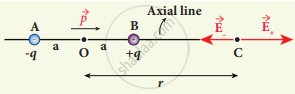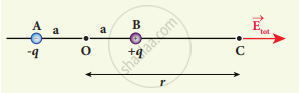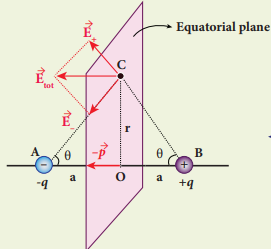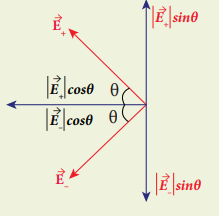Tamil Nadu Board of Secondary EducationHSC Science Class 12th

# Calculate the electric field due to a dipole on its axial line and equatorial plane. - Physics

Calculate the electric field due to a dipole on its axial line and equatorial plane.

#### Solution

Case (i):

Electric field due to an electric dipole at points on the axial line.

1. Consider an electric dipole placed on the x-axis A point C is located at a distance of r from the midpoint of the dipole along the axial line.Electric field of the dipole along the axial line
2. The electric field at a point C due to +q is
vec"E"_+ = 1/(4 pi ε_0) "q"/("r - a")^2 (along BC)
3. Since the electric dipole moment vector p is from -q to +q and is directed along BC, the above equation is rewritten as
vec"E"_+ = 1/(4 pi ε_0) "q"/("r - a")^2 hat"P"   ....(1)
4. 4. The electric field at a point C due to -q is
vec"E"_- = -1/(4 pi ε_0) "q"/("r + a")^2 hat"P"   ....(2)
5. Since +q is located closer to the point C than -q, vec"E"_+ is stronger than vec"E"_-.
Therefore, the length of the vec"E"_+ vector is drawn larger than that of vec"E"_- vector.
The total electric field at point C is calculated using the superposition principle of the electric field.
vec"E"_"tot" = vec"E"_+ + vec"E"_-
= 1/(4pi ε_0) "q"/("r - a")^2 hat"p" - 1/(4pi ε_0) "q"/("r + a")^2 hat"p"
vec"E"_"tot" = "q"/(4pi ε_0) (1/("r - a")^2 - 1/("r + a")^2)hat"p"  .....(3)
vec"E"_"tot" = "q"/(4pi ε_0) ("4ra"/("r"^2 - "a"^2)^2) hat"p"    .....(4)
r >> a
hence (r2 - a2)2 ≈ r4
Substitute in eqn (4)
vec"E"_"tot" = 1/(4pi ε_0)("4aq"/"r"^3)hat "p"
2"aq"hat"p" = vec"p"
vec"E"_"tot" = 1/(4pi ε_0) (2vac"p")/"r"^3
6. The direction of is shown in FigureTotal electric field of the dipole on the axial line

Case (ii)

1. Electric field due to an electric dipole at a point on the equatorial plane.
2. Consider a point C at a distance r from the midpoint 0 of the dipole on the equatorial plane.
3. Since point C is equidistant from +q and -q and are the same.
4. The direction of vec"E"_+ is along BC and the direction of vec"E"_- is along CA.
vec"E"_+ and vec"E"_- are resolved into two components; one component parallel to the dipole axis and the other perpendicular to it.
5. The perpendicular components |vec"E"| sinθ and |vec"E"_-| sinθ are oppositely directed and cancel each other.
"E"_"tot" = - |"E"_+| cos theta hat "p" - |vec"E"_-| cos theta hat "p"  .....(6)6. The magnitudes vec"E"_+ and vec"E"_- are the same and are given by
7. By substituting equation (7) into equation (6) we get
vec"E"_"tot" = - 1/(4piε_0) (2"q" cos theta)/("r"^2 + "a"^2) hat"p"
since cos θ = "a"/sqrt("r"^2 + "a"^2) hat
=- 1/(4piε_0) (2"qa")/("r"^2 +"a"^2)^(3/2) hat"p"
since vec"p" = "2qa"hat"p"   ....(8)
vec"E"_"tot" = - 1/(4piε_0) vec"p"/("r"^2 +"a"^2)^(3/2)|"E"_+| = |vec"E"_-| = 1/(4piε_0) "q"/("r"^2 + "a"^2)   .....(7)
8. At very large distances (r»a), the equation (8) becomes
vec"E"_"tot" = - 1/(4piε_0) vec"p"/"r"^3 (r >> a)
Concept: Electric Dipole and Its Properties
Is there an error in this question or solution?

#### APPEARS IN

Tamil Nadu Board Samacheer Kalvi Class 12th Physics Volume 1 and 2 Answers Guide
Chapter 1 Electrostatics
Evaluation | Q III. 4. | Page 74
Share